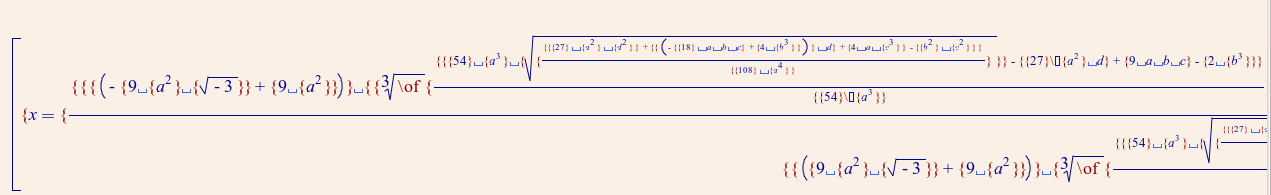# Maths - Cubic Functions

A cubic function has an x3 term, its general form is:

a x3 + b x2 + c x + d = 0

The three solutions are:

root1 = -a/3 + ((-2a3 + 9ab - 27c + sqrt((2a3 -9ab +27c)2 + 4(-a2 + 3b)3))/54)1/3 + ((-2a3 + 9ab - 27c - √((2a3 -9ab +27c)2 + 4(-a2 + 3b)3))/54)1/3

root2 = -a/3 - (1 + i sqrt(3))*0.5*((-2a3 + 9ab - 27c + sqrt((2a3 -9ab +27c)2 + 4(-a2 + 3b)3))/54)1/3 + (-1 + i sqrt(3))*0.5*((-2a3 + 9ab - 27c - sqrt((2a3 -9ab +27c)2 + 4(-a2 + 3b)3))/54)1/3

root3 = -a/3 + ((-2a3 + 9ab - 27c + sqrt((2a3 -9ab +27c)2 + 4(-a2 + 3b)3))/54)1/3 + ((-2a3 + 9ab - 27c - √((2a3 -9ab +27c)2 + 4(-a2 + 3b)3))/54)1/3

## Methods of solution

1. by factors
2. by completing the cobe
3. by formulae
4. by graph

### Sum and difference of two cubes

(a + b)(a2 - ab + b2)

= a (a2 - ab + b2) + b (a2 - ab + b2)

= a3 - a2b + ab2 + ba2 - ab2 + b3

= a3 + b3

simarly

(a - b)(a2 + ab + b2) = a3 - b3

## Using Symmetry

a x3 + b x2 + c x + d = 0

= 0

we can use the equation:

(x - x1)*(a' x2 + b' x + c') = 0

where x is our variable and x1, a', b' and c' are constants.

which has solutions at:

x = x1 andIn other words, can we use this to reduce the cubic to a quadratic in this way? First we have to multiply out the terms to see if its a general cubic:

(x - x1)*(a' x2 + b' x + c') = (a' x3 + b' x2 + c' x ) - x1*a' x2 - x1* b' x - x1* c')

= a' x3 + (b' - x1*a')x2 + (c' - x1* b') x - x1* c')

Therefore:

• a = a'
• b = b' - x1*a
• c = c' - x1* b'
• d = - x1* c'

So, puting this in terms of the original equation,

• a' = a
• b' = b + x1*a = b - d*a/c'
• c' = c + x1* b' = c - d*(b - d*a)/c'
• x1 = -d/c'

so:

c'2 - c*c' + d*(b - d*a) = 0

c' = (c± √(c2 - 4*d*(b - d*a)))/2

substituting in above gives:

• a' = a
• b' = b + x1*a = b - d*a/(c± √(c2 - 4*d*(b - d*a)))/2
• c' = (c± √(c2 - 4*d*(b - d*a)))/2
• x1 = -d/(c± √(c2 - 4*d*(b - d*a)))/2

## Program

There are a number of open source programs that can solve polynomial equations. I have used Axiom, how to install Axiom here.

To get a numeric solution for a given equation we can use complexSolve as shown here:

complexSolve(2*x^3+3*x^2+4*x+5 = 0,1.e-10)

I have put user input in red:

 (1) -> complexSolve(2*x^3+3*x^2+4*x+5 = 0,1.e-10) (1) [x= - 1.3711343313 043471426, x= - 0.0644328344 2475804354 9 - 1.3487610119 336750358 %i, x= - 0.0644328344 2475804354 9 + 1.3487610119 336750358 %i] Type: List Equation Polynomial Complex Float (2) ->

Or we can find a formula for, say, a quadratic equation using radicalSolve as shown here (unfortunately attempts to display large equations using fixed spacing do not really work so I have swiched the ouptut to LaTeX but its still too big):

 ```(3) -> )set output latex on (3) -> radicalSolve(a*x^3 + b*x^2 + c*x + d = 0,x)``` ``

## Code

Here is a Java function to return the cubic roots

```/**
This is incomplete and does not handle roots of -ve, let me know if you can help

code to solve cubic equation ax^3 + bx^2 + cx + d =0
result we be returned in resultReal,resultImaginary
to resultReal and resultImaginary
expects arrays to be setup before being called, like this:
double[] resultReal= new double;
double[] resultImaginary = new double;
*/
public void cubic(double a, double b, double c, double d,
double[] resultReal , double[] resultImaginary) {
if (Math.abs(a) < 0.000001) { // if a=0 equation is quadratic
// handle seperately to avoid divide by zero
return;
}
// recuring terms
double t1=(2*a*a*a -9*a*b +27*c);
double t2=(-a*a + 3*b);
double t3= t1*t1 + 4*t2*t2*t2;
if (t3<0) handle complex roots;
double t4=((-t1 + sqrt(t3))/54);
double t41=((-t1 - sqrt(t3))/54);
double t5=(-a*a + 3b);
double t6=sqrt(t1*t1 + 4*t5*t5*t5);
double t7=((-t1 - t6)/54);
resultReal = -a/3 + Math.pow(t4,1/3) + Math.pow(t7,1/3);
resultReal = -a/3 - 0.5*Math.pow(t4,1/3)+  -0.5*Math.pow(t4,1/3);
resultReal = -a/3 + Math.pow(t4,1/3) + Math.pow(t41,1/3)
resultImaginary = 0;
resultImaginary = sqrt(t3)*0.5*Math.pow(t4,1/3)+ sqrt(t3)*0.5*Math.pow(t4,1/3);
resultImaginary = 0;
return;
} ```

 metadata block see also: Correspondence about this page Book Shop - Further reading. Where I can, I have put links to Amazon for books that are relevant to the subject, click on the appropriate country flag to get more details of the book or to buy it from them.Mathematics for 3D game Programming - Includes introduction to Vectors, Matrices, Transforms and Trigonometry. (But no euler angles or quaternions). Also includes ray tracing and some linear & rotational physics also collision detection (but not collision response). Other Math Books Specific to this page here:

This site may have errors. Don't use for critical systems.